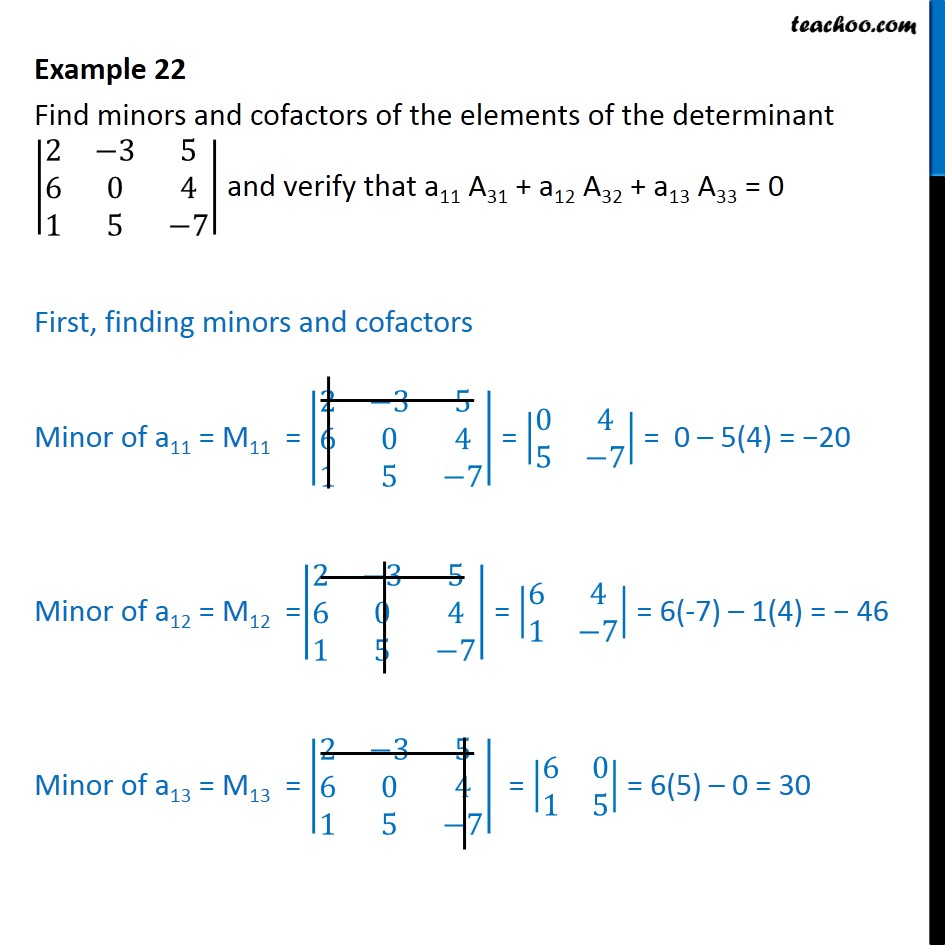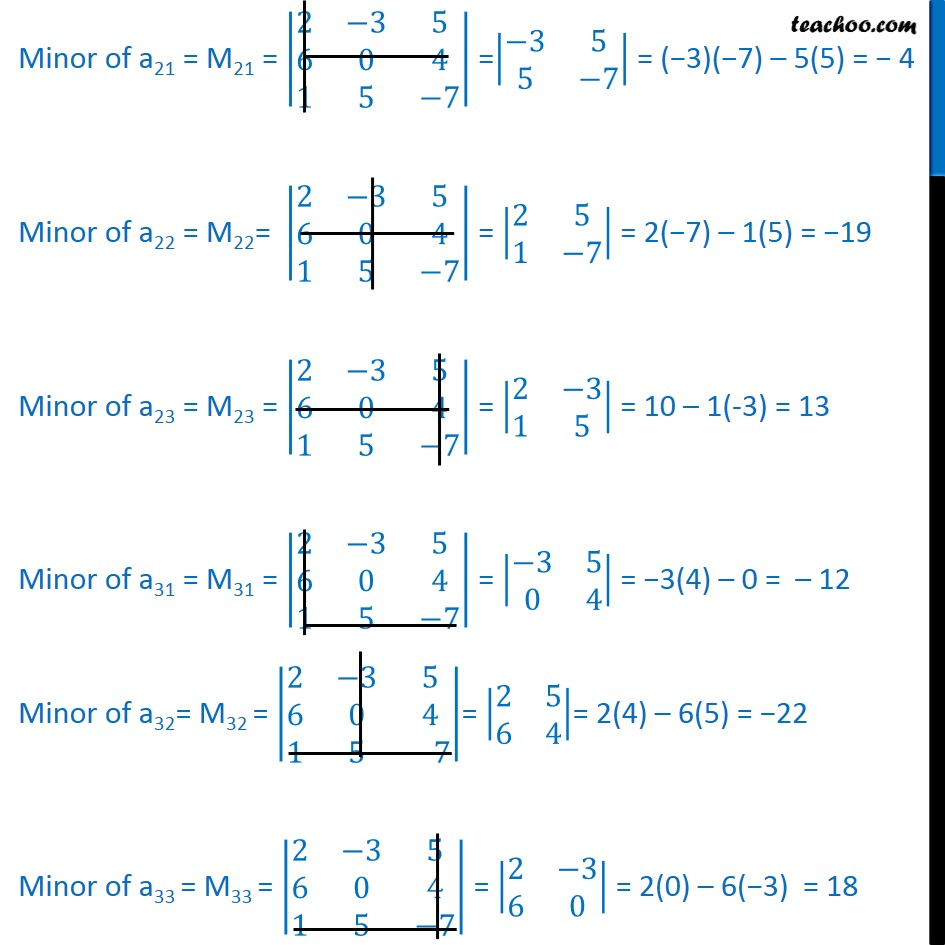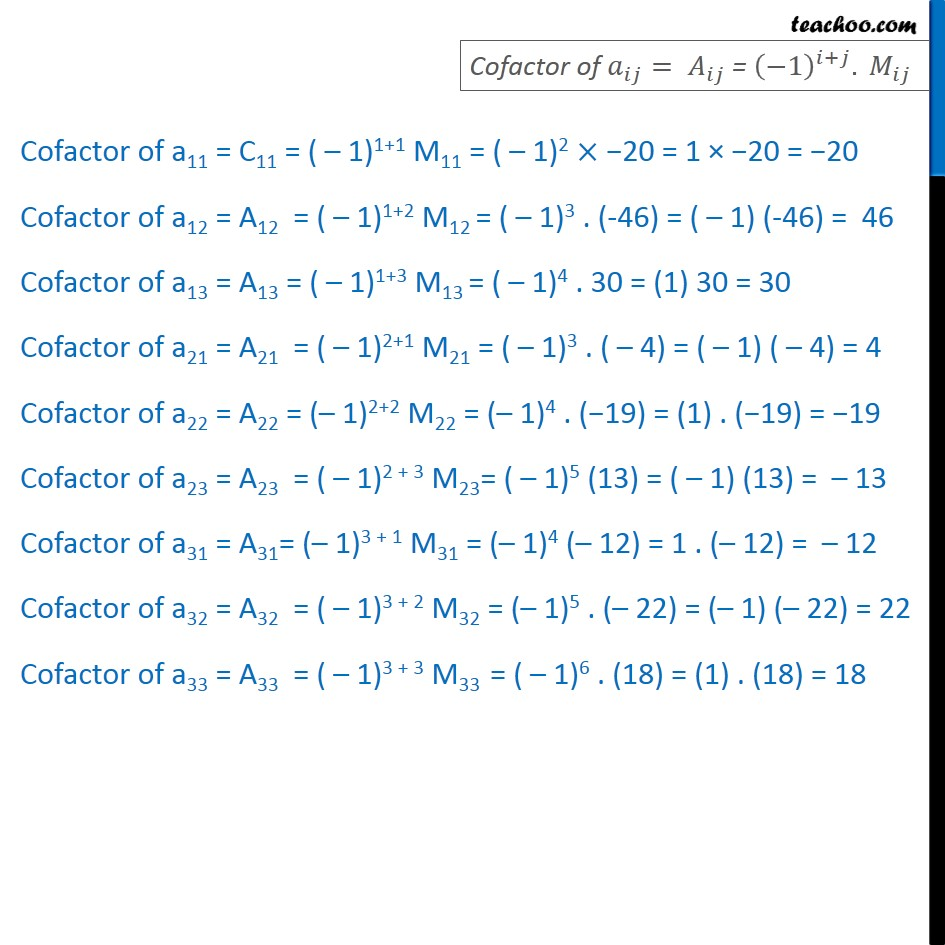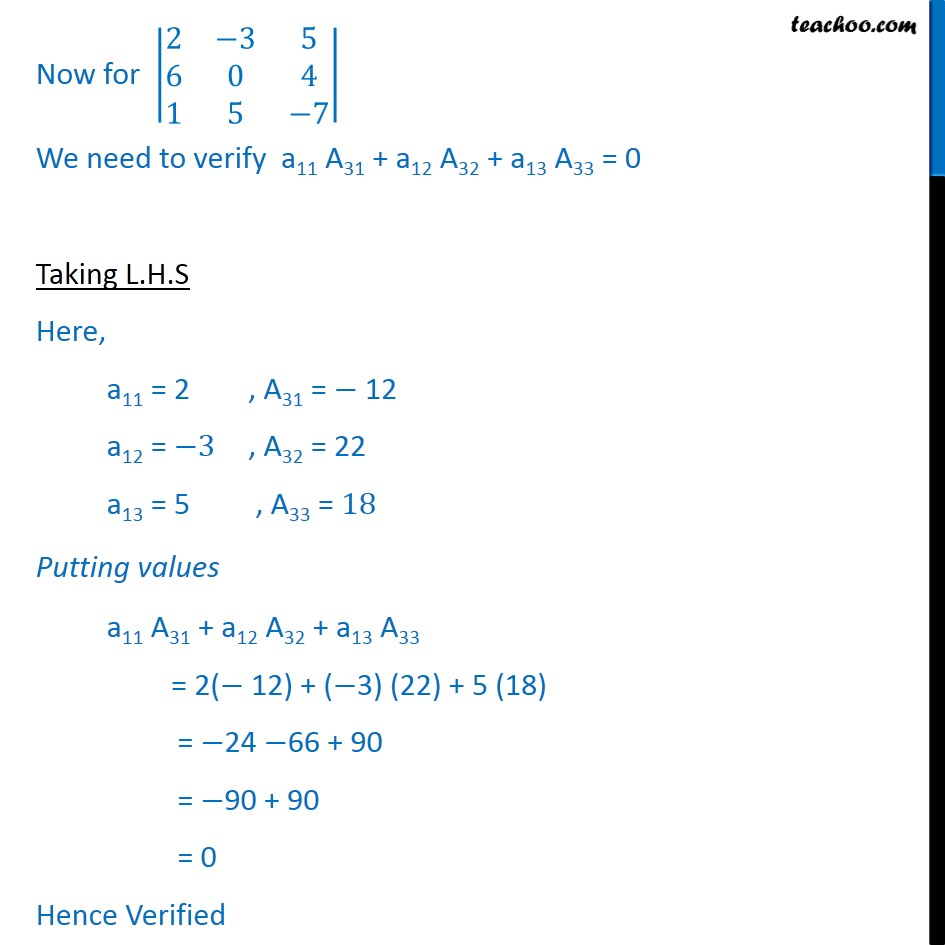Finding Minors and cofactors

Chapter 4 Class 12 Determinants
Concept wiseLearn in your speed, with individual attention - Teachoo Maths 1-on-1 Class

### Transcript

Example 11 Find minors and cofactors of the elements of the determinant 2 3 5 6 0 4 1 5 7 and verify that a11 A31 + a12 A32 + a13 A33 = 0 First, finding minors and cofactors Minor of a11 = M11 = 2 3 5 6 0 4 1 5 7 = 0 4 5 7 = 0 5(4) = 20 Minor of a12 = M12 = 2 3 5 6 0 4 1 5 7 = 6 4 1 7 = 6(-7) 1(4) = 46 Minor of a13 = M13 = 2 3 5 6 0 4 1 5 7 = 6 0 1 5 = 6(5) 0 = 30 Minor of a21 = M21 = 2 3 5 6 0 4 1 5 7 = 3 5 5 7 = ( 3)( 7) 5(5) = 4 Minor of a22 = M22= 2 3 5 6 0 4 1 5 7 = 2 5 1 7 = 2( 7) 1(5) = 19 Minor of a23 = M23 = 2 3 5 6 0 4 1 5 7 = 2 3 1 5 = 10 1(-3) = 13 Minor of a31 = M31 = 2 3 5 6 0 4 1 5 7 = 3 5 0 4 = 3(4) 0 = 12 Minor of a32= M32 = 2 3 5 6 0 4 1 5 7 = 2 5 6 4 = 2(4) 6(5) = 22 Minor of a33 = M33 = 2 3 5 6 0 4 1 5 7 = 2 3 6 0 = 2(0) 6( 3) = 18 Cofactor of a11 = C11 = ( 1)1+1 M11 = ( 1)2 20 = 1 20 = 20 Cofactor of a12 = A12 = ( 1)1+2 M12 = ( 1)3 . (-46) = ( 1) (-46) = 46 Cofactor of a13 = A13 = ( 1)1+3 M13 = ( 1)4 . 30 = (1) 30 = 30 Cofactor of a21 = A21 = ( 1)2+1 M21 = ( 1)3 . ( 4) = ( 1) ( 4) = 4 Cofactor of a22 = A22 = ( 1)2+2 M22 = ( 1)4 . ( 19) = (1) . ( 19) = 19 Cofactor of a23 = A23 = ( 1)2 + 3 M23= ( 1)5 (13) = ( 1) (13) = 13 Cofactor of a31 = A31= ( 1)3 + 1 M31 = ( 1)4 ( 12) = 1 . ( 12) = 12 Cofactor of a32 = A32 = ( 1)3 + 2 M32 = ( 1)5 . ( 22) = ( 1) ( 22) = 22 Cofactor of a33 = A33 = ( 1)3 + 3 M33 = ( 1)6 . (18) = (1) . (18) = 18 Now for 2 3 5 6 0 4 1 5 7 We need to verify a11 A31 + a12 A32 + a13 A33 = 0 Taking L.H.S Here, a11 = 2 , A31 = 12 a12 = 3 , A32 = 22 a13 = 5 , A33 = 18 Putting values a11 A31 + a12 A32 + a13 A33 = 2( 12) + ( 3) (22) + 5 (18) = 24 66 + 90 = 90 + 90 = 0 Hence Verified